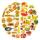# Multiplication principle + real numbers - math problems

#### Number of problems found: 1

• EventsEvent P has probability of 0.84. What is the probability that the event P occurs in 3, 5, 7 try.

We apologize, but in this category are not a lot of examples.
Do you have an interesting mathematical word problem that you can't solve it? Submit a math problem, and we can try to solve it.

We will send a solution to your e-mail address. Solved examples are also published here. Please enter the e-mail correctly and check whether you don't have a full mailbox.

Please do not submit problems from current active competitions such as Mathematical Olympiad, correspondence seminars etc...